Circles in Circles

The following pictures show n unit circles packed inside the smallest known circle (of radius r).

 1.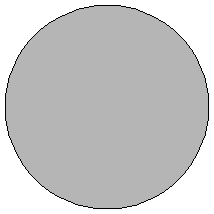2.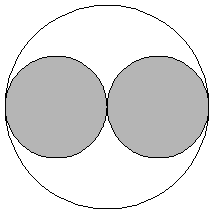3.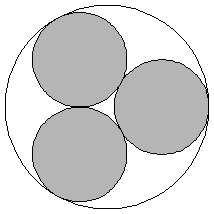r = 1Trivial. r = 2Trivial. r = 1 + 2 / √3 = 2.154+Trivial.

 4.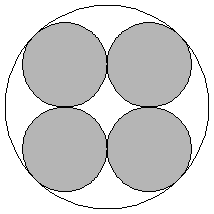5.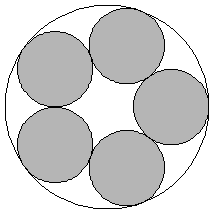6.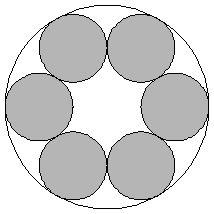r = 1 + √2 = 2.414+Trivial. r = 2.701+Proved by Graham in 1968. r = 3Proved by Graham in 1968.

 7.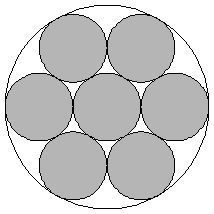8.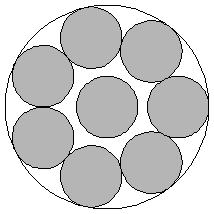9.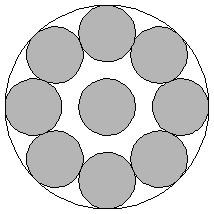r = 3Proved by Graham in 1968. r = 3.304+Proved by Braaksma in 1963. r = 3.613+Proved by Pirl in 1969.

 10.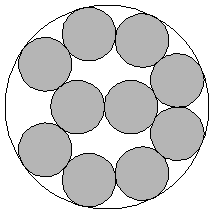11.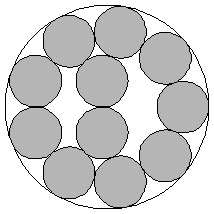12.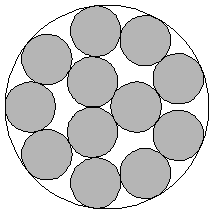r = 3.813+Proved by Pirl in 1969. r = 3.923+Proved by Melissen in 1994. r = 4.029+Found by Kravitz in 1967.

 13.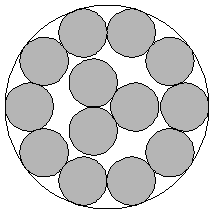14.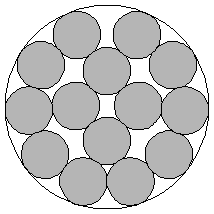15.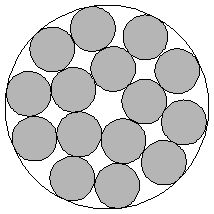r = 2 + √5 = 4.236+Proved by F. Fodor in 2003. r = 4.328+Found by Goldberg in 1971. r = 4.521+Found by Kravitz in 1967.

 16.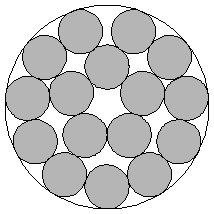17.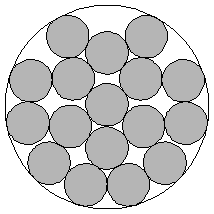18.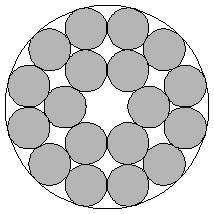r = 4.615+Found by Goldberg in 1971. r = 4.792+Found by Reis in 1975. r = 1 + √2 + √6 = 4.863+ Found by Kravitz in 1967.

 19.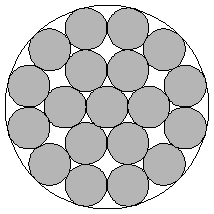20.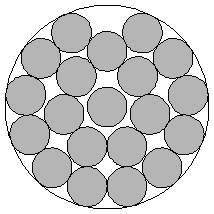r = 1 + √2 + √6 = 4.863+Proved by F. Fodor in 1999. r = 5.122+Found by Goldberg in 1971.